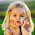## JAVA95

Let's learn java programming language with easy steps

# String Programming Interview Questions in Java

### String Programming Interview Questions

Here we are gonna to discuss some string programming interview questions in java. String handling is the most important topic in core java.

In this article you will see most frequently asked java string programming interview questions and answers.

Let's start java string programs asked in interview one-by-one.

### (1) What is the output of following program?

class Test1
{
public static void main(String args[])
{
String a = "javatutorial95"+100+200;
System.out.println(a);
}
}

Output: javatutorial95100200

### (2) What is the output of following program?

class Test2
{
public static void main(String args[])
{
String a = 10+20+"javatutorial95"+20+30;
System.out.println(a);
}
}

Output: 30javatutorial952030

### (3) Write a program to reverse a String in java without using String API?

class Test3
{
public static void main(String args[])
{
String s = "Best Country";
String reverse = "";
for(int i = s.length()-1; i>=0; --i)
{
reverse +=s.charAt(i);
}
System.out.println(reverse);
}
}

Output: yrtnuoC tseB

### (4) Write a java program to check String is palindrome or not?

class Test4
{
public static void main(String args[])
{
String name = "MADAM";//String to be checked for palindrom
String reverse = "";
for(int i = name.length()-1; i>=0; --i)
{
reverse += name.charAt(i);
}
System.out.println(reverse);

if(reverse.equalsIgnoreCase(name))
{
System.out.println("String is palindrome");
}
else
{
System.out.println("String is not palindrome");
}
}
}

String is plindrome

### (5) Write a java program to find the duplicate words and their number of occurrences in a string?

import java.util.*;
class Test5
{
static void duplicateWords(String string1)
{
//splitting string1 into words
String[] words = string1.split(" ");

//creating HashMap with word as key and count as value
HashMap<String, Integer> wordcount = new HashMap<String, Integer>();

//checking each words
for(String word : words)
{
if(wordcount.containsKey(word.toLowerCase()))
{
wordcount.put(word.toLowerCase(), wordcount.get(word.toLowerCase())+1);
}
else
{
wordcount.put(word.toLowerCase(), 1);
}
}
Set<String> wordsstring = wordcount.keySet();
for(String word : wordsstring)
{
if(wordcount.get(word)>1)
{
System.out.println(word+"  :  "+wordcount.get(word));
}
}
}

public static void main(String args[])
{
duplicateWords(dog is Dog not cat);
duplicateWords(cat is Dog not cat and cat);
}
}

Output: dog : 2
cat : 3

### (6) Write a java program to count the number of words in a string?

import java.util.*;
class Test6
{
public static void main(String args[])
{
//using Scanner class
Scanner s = new Scanner(System.in);
String s1 = s.nextLine();
String[] words = s1.trim().split(" ");
System.out.println("Number of word in a string "+words.length);
}
}

### (7) Write a java program to count the number of occurrences of each characters in string?

Occurrence of characters in string e.g "javatutorial" j = 1, a = 3, v = 1..

import java.util.*;
class Test7
{
static void characterCount(String string1)
{
HashMap<Character, Integer> hm = new HashMap<Character, Integer>();
//converting given string to char array
char[] ch = string1.toCharArray();
//checking eahc char of ch
for(char c : ch)
{
if(hm.containsKey(c))
{
hm.put(c, hm.get(c)+1);
}
else
{
hm.put(c, 1);
}
}
System.out.println(hm);
}
public static void main(String args[])
{
characterCount("India is a good country");
}
}

### (8) How to check total number of vowels in a java string?

There are 5 vowels in a to z alphabet e.g 'a', 'e', 'i', 'o', 'u'.

import java.util.*;
class Test8
{
public static void main(String args[])
{
System.out.println("Enter some string");
Scanner sc = new Scanner(System.in);
String str = sc.nextLine();
char chars[] = str.toCharArray();
int count = 0;
for(char c : chars)
{
switch(c)
{
case 'a':
case 'e':
case 'i':
case 'o':
case 'u':
count++;
break;
}
}
System.out.println("Number of vowels in string "+count);
}
}

Output: Enter some string
i love you
Number of vowels in string 5

### (9) How to sort the string without using string API in java?

class Test9
{
public static void main(String args[])
{
String original = "zfabcd";
int j = 0;
char temp = 0;
char chars[] = original.toCharArray();
for(int i = 0; i<chars.length; i++)
{
for(j = 0; j<chars.length; j++)
{
if (chars[j]>chars[i])
{
temp = chars[i];
chars[i]=chars[j];
chars[j]=temp;
}
}
}
for(int k = 0; k<chars.length; k++)
{
System.out.println(chars[k]);
}
}
}

Output: a
b
c
d
f
z

Above all the java string programs examples are quite useful in any core java interview.

Share:

1.Please provide some array based interview questions

2.Hi Anurag,

I love all the posts, I really enjoyed.

I want to make a tool that does the 2 following things::

- Open files that are not .txt but can be opened as .txt and return them as a string. It just returns an empty string at the moment.
- The filenames are unknown, just the file extension at the end and the the YYYYMMDD number in front are always the same, therefore I'd like the app to simply scan every file in the same folder (not the same file twice, obviously). How can this be done?

Thank you very much and will look for more postings from you.

Thank you,
Irene Hynes